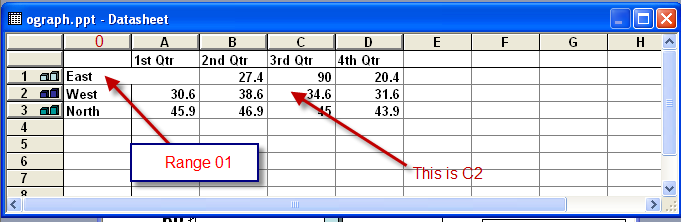PowerPoint tips, hints and tutorials that will change your presentations for ever!

INDEXVBA to Modify MSGraph in PowerPoint

This is a routine that finds MSGraph objects in powerPoint and modifies them as required. It will not work with the Excel graph format used in PPT 2007.

First let's look at a DataSheet because you need to understand the way it is referenced by RangeThe first colimn which is unlabelled is column "0". The cells are referred to by Column+Row so C2 arrowed is column C , Row 2.

Here is the code used to modify all the graphs in a presentation.

Sub ograph()
Dim osld As Slide
Dim oshp As Shape
Dim ograph As Object
For Each osld In ActivePresentation.Slides
'Find the msGraph object
For Each oshp In osld.Shapes
If oshp.Type = msoEmbeddedOLEObject Then
If oshp.OLEFormat.ProgID Like "MSGraph*" Then
'it's a graph
Set ograph = oshp.OLEFormat.Object

'do something with it
'eg swap the Title

If ograph.HasTitle Then
If ograph.ChartTitle.Text = "2007" Then ograph.ChartTitle.Text = "2008"
End If

'or change the data

If ograph.Application.DataSheet.Range("01").Value = "East" Then _
ograph.Application.DataSheet.Range("01").Value = "NEWVALUE"

End If 'it's an OLE object
End If 'it's a graph

Next oshp
Next osld
End Sub

Confusingly if you reference by Cells the numbering is different:

Range "A2" for example is Cells(4,3)- (4th Row , 3rd Column)

Code for global replace using Cells

This will search the whole data sheet. Alter strReplace and strWith to suit. As written it will only search the first row. To search the whole sheet comment IN the four lines of code in green starting with 'Do While ......

Don't know what to do with the code - See here.

```Sub ograph()
Dim osld As Slide
Dim oshp As Shape
Dim ograph As Object
Dim Icol As Integer
Dim Irow As Integer
Dim strReplace As String
Dim strWith As String
For Each osld In ActivePresentation.Slides
'Find the msGraph object
For Each oshp In osld.Shapes
If oshp.Type = msoEmbeddedOLEObject Then
If oshp.OLEFormat.ProgID Like "MSGraph*" Then
'it's a graph
Set ograph = oshp.OLEFormat.Object
'do something with it
Irow = 1
Icol = 2 ' avoids blank cell at 1,1
strReplace = "Qtr"
strWith = "Quarter"
'Do While ograph.Application.DataSheet.Cells(Irow, Icol) <> ""
Do While ograph.Application.DataSheet.Cells(Irow, Icol) <> ""
ograph.Application.DataSheet.Cells(Irow, Icol) = _
Replace(ograph.Application.DataSheet.Cells(Irow, Icol), strReplace, strWith)
Icol = Icol + 1
Loop
'Icol = 1
'Irow = Irow + 1
'Loop
End If 'it's an OLE object
End If 'it's a graph
Next oshp
Next osld
End Sub ```

Back to the Index Page